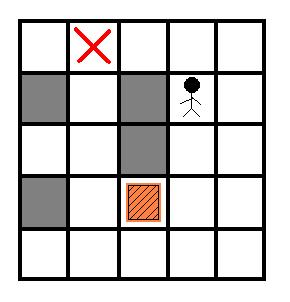# ACM暑假集训（Ⅲ）搜索 A-A / HDU 1254 ：推箱子 （BFS）Input

Output

Sample Input
1
5 5
0 3 0 0 0
1 0 1 4 0
0 0 1 0 0
1 0 2 0 0
0 0 0 0 0

Sample Output
4

AC代码：

#include<iostream>
using namespace std;
#include<cstring>
#include<queue>
int a,M,N;
struct student{
int x,y,bx,by,num;
friend bool operator<(student s1,student s2){
return s1.num>s2.num;
}
}star;
bool mark;
int bfs(){
int dx={0,0,1,-1},dy={1,-1,0,0};
student now,next;
priority_queue <student> p;
p.push(star);
mark[star.x][star.y][star.bx][star.by] = true;
while(!p.empty()){
now=p.top();
p.pop();
if(a[now.bx][now.by]==3) return now.num;
for(int i=0;i<4;i++){
int x=now.x+dx[i];
int y=now.y+dy[i];

if(a[x][y]==1||x<0||x>=M||y<0||y>=N) continue;
next.x=x;
next.y=y;
next.bx=now.bx;
next.by=now.by;
next.num=now.num;
if(x==now.bx&&y==now.by){//如果下个点是箱子的位置
int xx=x+dx[i],yy=y+dy[i];//搬运工此时只能从走过来的那一个方向推箱子（不用for i:0 ~ 4）
if(a[xx][yy]==1||xx<0||xx>=M||yy<0||yy>=N) continue;                        //墙 继续    //越界 继续
next.bx=xx;
next.by=yy;
next.num++;
}
if (mark[x][y][next.bx][next.by])//如果推完后的状态已经经历过就不推了
continue;
mark[x][y][next.bx][next.by] = true;
p.push(next);
}
}
return -1;
}

int main()
{
int T;
cin>>T;
while(T--){
memset(mark, 0, sizeof(mark));                     //初始化不能忘记，否则wa
cin>>M>>N;
for(int i=0;i<M;i++){
for(int j=0;j<N;j++){
cin>>a[i][j];
if(a[i][j]==4){
star.x=i;
star.y=j;
star.num=0;
a[i][j]=0;
}
if(a[i][j]==2){
star.bx=i;
star.by=j;
a[i][j]=0;
}
}
}
cout<<bfs()<<endl;
}
}


09-25190
07-2663904-292220
03-183867
12-151023
02-25445
07-12723
07-21935
09-19762
10-30739
05-03659
12-113171
06-09788
01-17107
04-27360
09-05860
11-06841
03-08189